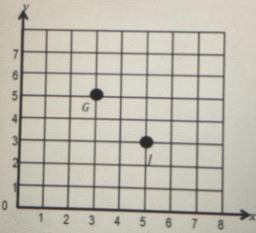# Garrett

Garrett and Jeffrey are planning a treasure hunt. They decide to place a treasure at a point that is a distance of 5 units from the x-axis and three units from the y -axis. Jeffrey places a treasure at point J at coordinates (5,3), and Garrett places one at point G=(3,5). Who put the treasure in the right place? Explain how you know.

Result

w = (Correct answer is: G)### Step-by-step explanation:Did you find an error or inaccuracy? Feel free to write us. Thank you!

Tips for related online calculators
Line slope calculator is helpful for basic calculations in analytic geometry. The coordinates of two points in the plane calculate slope, normal and parametric line equation(s), slope, directional angle, direction vector, the length of the segment, intersections of the coordinate axes, etc.